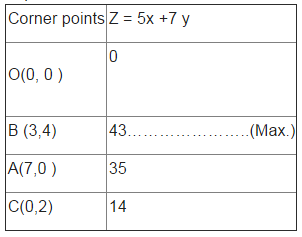Courses

# Test: Linear Programming (CBSE Level) - 1

## 25 Questions MCQ Test Mathematics (Maths) Class 12 | Test: Linear Programming (CBSE Level) - 1

Description
This mock test of Test: Linear Programming (CBSE Level) - 1 for JEE helps you for every JEE entrance exam. This contains 25 Multiple Choice Questions for JEE Test: Linear Programming (CBSE Level) - 1 (mcq) to study with solutions a complete question bank. The solved questions answers in this Test: Linear Programming (CBSE Level) - 1 quiz give you a good mix of easy questions and tough questions. JEE students definitely take this Test: Linear Programming (CBSE Level) - 1 exercise for a better result in the exam. You can find other Test: Linear Programming (CBSE Level) - 1 extra questions, long questions & short questions for JEE on EduRev as well by searching above.
QUESTION: 1

### In a LPP, the objective function is always

Solution:

In a LPP, the objective function is always linear.

QUESTION: 2

### Maximize Z = – x + 2y, subject to the constraints: x ≥ 3, x + y ≥ 5, x + 2y ≥ 6, y ≥ 0.

Solution:

Objective function is Z = - x + 2 y ……………………(1).
The given constraints are : x ≥ 3, x + y ≥ 5, x + 2y ≥ 6, y ≥ 0.

Corner points Z =  - x + 2y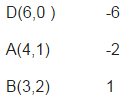Here , the open half plane has points in common with the feasible region .
Therefore , Z has no maximum value.

QUESTION: 3

### Two godowns A and B have grain capacity of 100 quintals and 50 quintals respectively. They supply to 3 ration shops, D, E and F whose requirements are 60, 50 and 40 quintals respectively. The cost of transportation per quintal from the godowns to the shops are given in the following table: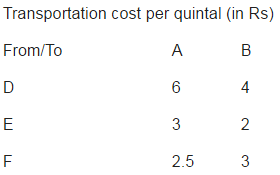How should the supplies be transported in order that the transportation cost is minimum? What is the minimum cost?

Solution:

Let the number of units of grain transported from godown A to D = x And the number of units of grain transported from godown A to E = y Therefore , the number of units of grain transported from godown A to F = 100 – (x+y) Therefore , the number of units of grain transported from godown B to D = 60 – x The number of units of grain transported from godown B to E = 50 – y The number of units of grain transported from godown B to F = x + y – 60 . Here , the objective function is :Minimise Z = 2.5x + 1.5y + 410 . , subject to constraints : 60 - x ≥ 0,50 - y ≥ 0 ,100 – (x + y) ≥ 0 , (x + y) - 60 ≥ 0 , x,y ≥ 0.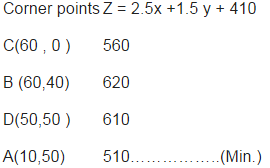Here Z = 510 is minimum.i.e. From A : 10,50, 40 units; From B: 50,0,0 units to D, E and F respectively and minimum cost = Rs 510 .

QUESTION: 4

Corner points of the feasible region for an LPP are (0, 2), (3, 0), (6, 0), (6, 8) and (0, 5). Let F = 4x + 6y be the objective function. Maximum of F – Minimum of F =

Solution:

Here the objective function is given by : F = 4x +6y .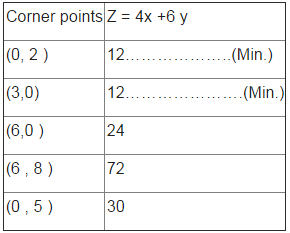Maximum of F – Minimum of F = 72 – 12 = 30 .

QUESTION: 5

A linear programming problem is one that is concerned with

Solution:

A linear programming problem is one that is concerned with finding the optimal value (maximum or minimum) of a linear function of several variables .

QUESTION: 6

Which of the following types of problems cannot be solved by linear programming methods

Solution:

Traffic signal control types of problems cannot be solved by linear programming methods .

QUESTION: 7

The optimal value of the objective function Z = ax + by may or may not exist, if the feasible region for a LPP is

Solution:

The optimal value of the objective function Z = ax + by may or may not exist, if the feasible region for a LPP is unbounded.

QUESTION: 8

One kind of cake requires 200g of flour and 25g of fat, and another kind of cake requires 100g of flour and 50g of fat. Find the maximum number of cakes which can be made from 5kg of flour and 1 kg of fat assuming that there is no shortage of the other ingredients used in making the cakes.

Solution:

Let number of cakes of first type = x
And number of cakes of second type = y
Therefore , the above L.P.P. is given as :
Minimise , Z = x +y , subject to the constraints : 200x +100y ≤ 5000 and. 25x +50y ≤ 1000, i.e. 2x + y ≤ 50 and x +2y ≤ 40 x, y ≥ 0.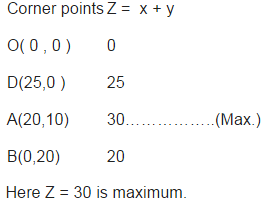i.e Maximum number of cakes = 30 , 20 of kind one and 10 cakes of another kind .

QUESTION: 9

An oil company has two depots A and B with capacities of 7000 L and 4000 L respectively. The company is to supply oil to three petrol pumps, D, E and F whose requirements are 4500L, 3000L and 3500L respectively. The distances (in km) between the depots and the petrol pumps is given in the following table: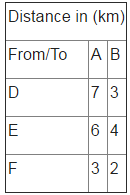Assuming that the transportation cost of 10 litres of oil is Re 1 per km, how should the delivery be scheduled in order that the transportation cost is minimum?What is the minimum cost?

Solution:

Here objective function is : Z = 3x + y + 39500 , subject to constraints : : x + y ≤ 7000, x ≤ 4500, x + y ≥ 3500, , y ≤ 3000x , x,y ≥ 0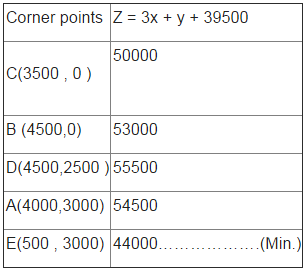Here Z = 4400 is minimum.i.e. . From A: 500, 3000 and 3500 litres; From B: 4000, 0, 0 litres to D, E and F respectively; Minimum cost = Rs 4400 .

QUESTION: 10

Corner points of the feasible region determined by the system of linear constraints are (0, 3), (1, 1) and (3, 0). Let Z = px+qy, where p, q > 0. Condition on p and q so that the minimum of Z occurs at (3, 0) and (1, 1) is

Solution:

We have Z = px + qy , At (3, 0) Z = 3p ……………………………….(1) At (1 , 1) Z = p + q …………………………(2) Therefore , from (1) and (2) : We have : p = q/2 .

QUESTION: 11

In linear programming feasible region (or solution region) for the problem is

Solution:

In linear programming feasible region (or solution region) for the problem is given by The common region determined by all the constraints including the non – negative constraints x ⩾0, y ⩾ 0

QUESTION: 12

In a LPP if the objective function Z = ax + by has the same maximum value on two corner points of the feasible region, then every point on the line segment joining these two points give the same

Solution:

In a LPP if the objective function Z = ax + by has the same maximum value on two corner points of the feasible region, then every point on the line segment joining these two points give the same maximum value .

QUESTION: 13

A factory makes tennis rackets and cricket bats. A tennis racket takes 1.5 hours of machine time and 3 hours of craftman’s time in its making while a cricket bat takes 3 hour of machine time and 1 hour of craftman’s time. In a day, the factory has the availability of not more than 42 hours of machine time and 24 hours of craftsman’s time. What number of rackets and bats must be made if the factory is to work at full capacity?

Solution:

Let number of rackets made = x
And number of bats made = y
Therefore , the above L.P.P. is given as :
Maximise , Z = x +y , subject to the constraints : 1.5x +3y ≤ 42 and. 3x +y ≤ 24, i.e.0.5x + y ≤ 14 i.e. x +2y ≤ 28 and 3x +y ≤ 24 , x, y ≥ 0.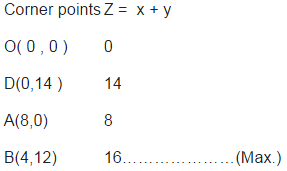Here Z = 16 is maximum. i.e Maximum number of rackets = 4 and number of bats = 12.

QUESTION: 14

A fruit grower can use two types of fertilizer in his garden, brand P and brand Q. The amounts (in kg) of nitrogen, phosphoric acid, potash, and chlorine in a bag of each brand are given in the table. Tests indicate that the garden needs at least 240 kg of phosphoric acid, at least 270 kg of potash and at most 310 kg of chlorine.If the grower wants to minimise the amount of nitrogen added to the garden, how many bags of each brand should be used? What is the minimum amount of nitrogen added in the garden? should the delivery be scheduled in order that the transportation cost is minimum?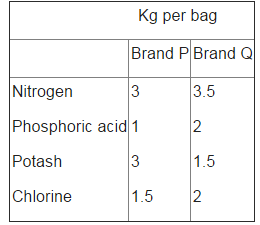Solution:

Let the number of bags used for fertilizer of brand P = x And the number of bags used for fertilizer of brand Q = y . Here , Z = 3x + 3.5y subject to constraints : :1.5 x +2 y ≤ 310, x + 2y ≥ 240, 3x + 1.5y ≥ 270 , x,y ≥ 0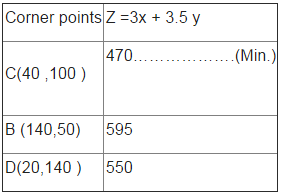Here Z = 470 is minimum i.e. 40 bags of brand P and 100 bags of brand Q; Minimum amount of nitrogen = 470 kg.

QUESTION: 15

In a LPP, the linear inequalities or restrictions on the variables are called

Solution:

In a LPP, the linear inequalities or restrictions on the variables are called Linear constraints

QUESTION: 16

In linear programming infeasible solutions

Solution:

In linear programming infeasible solutions fall outside the feasible region .

QUESTION: 17

In linear programming problems the function whose maxima or minima are to be found is called

Solution:

In linear programming problems the function whose maxima or minima are to be found is called Objective function .

QUESTION: 18

A factory makes tennis rackets and cricket bats. A tennis racket takes 1.5 hours of machine time and 3 hours of craftman’s time in its making while a cricket bat takes 3 hour of machine time and 1 hour of craftman’s time. In a day, the factory has the availability of not more than 42 hours of machine time and 24 hours of craftsman’s time. If the profit on a racket and on a bat is Rs 20 and Rs 10 respectively, find the maximum profit of the factory when it works at full capacity?

Solution:

Let number of rackets made = x
And number of bats made = y
Therefore , the above L.P.P. is given as :
Maximise , Z = x +y , subject to the constraints : 1.5x +3y ≤ 42 and. 3x +y ≤ 24, i.e.0.5x + y ≤ 14 i.e. x +2y ≤ 28 and 3x +y ≤ 24 , x, y ≥ 0.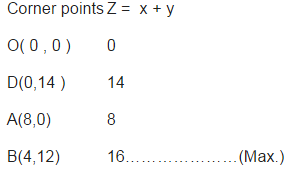Here Z = 16 is maximum. i.e Maximum number of rackets = 4 and number of bats = 12.
Here , profit function is P = 20x + 10y
Profit is maximum at x = 4 and y = 12 .
Therefore , maximum profit = 20(4) + 10 ( 12) = 200.i.e. Rs.200.

QUESTION: 19

A fruit grower can use two types of fertilizer in his garden, brand P and brand Q. The amounts (in kg) of nitrogen, phosphoric acid, potash, and chlorine in a bag of each brand are given in the table. Tests indicate that the garden needs at least 240 kg of phosphoric acid, at least 270 kg of potash and at most 310 kg of chlorine.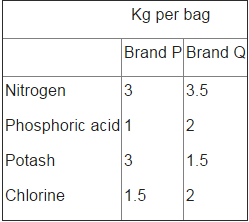If the grower wants to maximise the amount of nitrogen added to the garden, how many bags of each brand should be added? What isthe maximum amount of nitrogen added?

Solution:

Let the number of bags used for fertilizer of brand P = x And the number of bags used for fertilizer of brand Q = y . Here , Z = 3x + 3.5y subject to constraints : :1.5 x +2 y ≤ 310, x + 2y ≥ 240, 3x + 1.5y ≥ 270 , x,y ≥ 0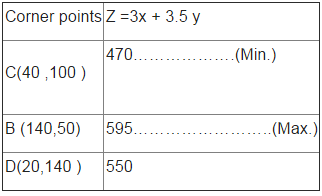Here Z = 595 is maximum i.e. 140 bags of brand P and 50 bags of brand Q; Maximum amount of nitrogen = 595 kg .

QUESTION: 20

Determine the maximum value of Z = 3x + 4y if the feasible region (shaded) for a LPP is shown in Figure above.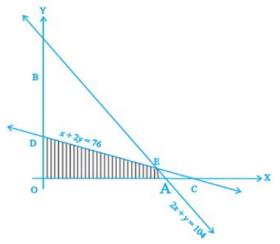Solution: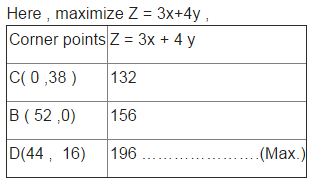QUESTION: 21

In linear programming, optimal solution

Solution:

In linear programming, optimal solution satisfies all the constraints as well as the objective function .

QUESTION: 22

In linear programming problems the optimum solution

Solution:

In linear programming problems the optimum solution satisfies a set of linear inequalities (called linear constraints) .

QUESTION: 23

A manufacturer produces nuts and bolts. It takes 1 hour of work on machine A and 3 hours on machine B to produce a package of nuts. It takes 3 hours on machine A and 1 hour on machine B to produce a package of bolts. He earns a profit of Rs17.50 per package on nuts and Rs 7.00 per package on bolts. How many packages of each should be produced each day so as to maximise his profit, if he operates his machines for at the most 12 hours a day?

Solution:

Let number of packages of nuts produced = x
And number of packages of bolts produced = y
Therefore , the above L.P.P. is given as :
Maximise , Z = 17.50x +7y , subject to the constraints : x +3y ≤ 12 and. 3x +y ≤ 12, x, y ≥ 0.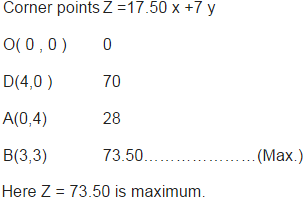i.e 3 packages of nuts and 3 packages of bolts;
Maximum profit = Rs 73.50.

QUESTION: 24

A toy company manufactures two types of dolls, A and B. Market tests and available resources have indicated that the combined production level should not exceed 1200 dolls per week and the demand for dolls of type B is at most half of that for dolls of type A. Further, the production level of dolls of type A can exceed three times the production of dolls of other type by at most 600 units. If the company makes profit of Rs 12 and Rs 16 per doll respectively on dolls A and B, how many of each should be produced weekly in order to maximise the profit?

Solution:

Here , Maximise Z = 12x + 16y , subject to constraints : : x + y ≤ 1200, x - 2y ≥ 0, x - 3y ≤ 600 , x,y ≥ 0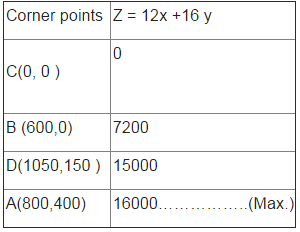Here Z = 16000 is maximum.i.e. 800 dolls of type A and 400 dolls of type B; Maximum profit = Rs 16000 .

QUESTION: 25

Feasible region (shaded) for a LPP is shown in Figure. Maximize Z = 5x + 7y.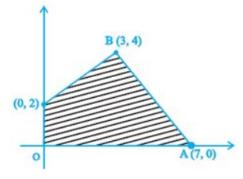Solution: